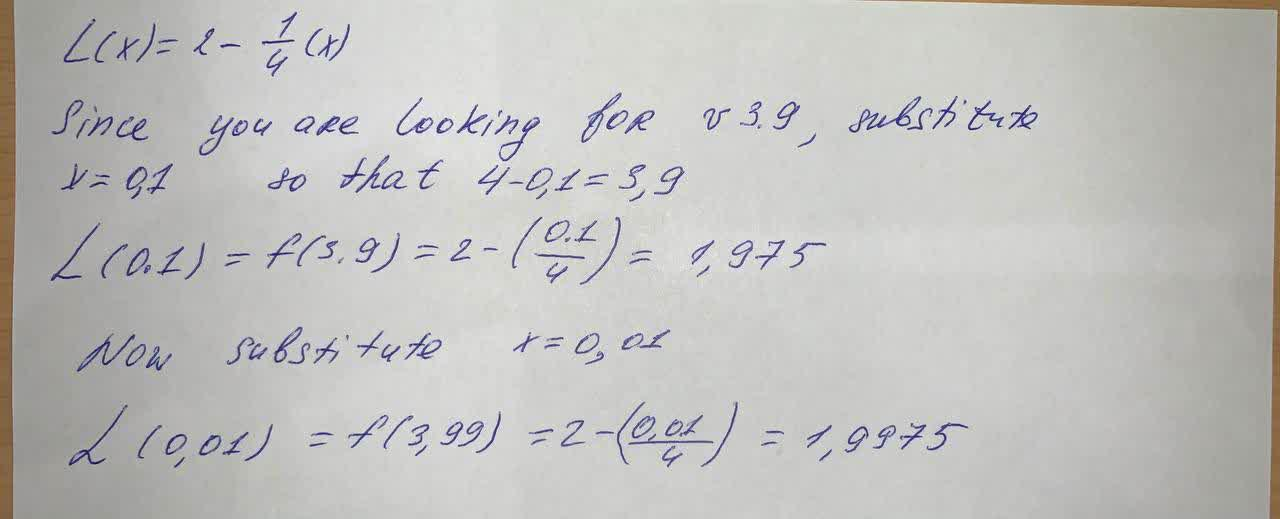abondantQ

2021-06-01

Find the linear approximation of the function $f\left(x\right)=\sqrt{4-x}$ at $a=0$
Use L(x) to approximate the numbers $\sqrt{3.9}$ and $\sqrt{3.99}$ Round to four decimal placesestenutC

ExpertJeffrey Jordon

Expert

$L\left(x\right)=2-\frac{1}{4}\left(x\right)$

Since you are looking for v 3.9, substiute $x=0,1$ so that $4-0,1=3,9$

$L\left(0,1\right)=f\left(3.9\right)=2-\left(\frac{0.1}{4}\right)=1.975$

Now substitute $x=0,01$

$L\left(0,01\right)=f\left(3,99\right)=2-\left(\frac{0.01}{4}\right)=1.9975$

Do you have a similar question?